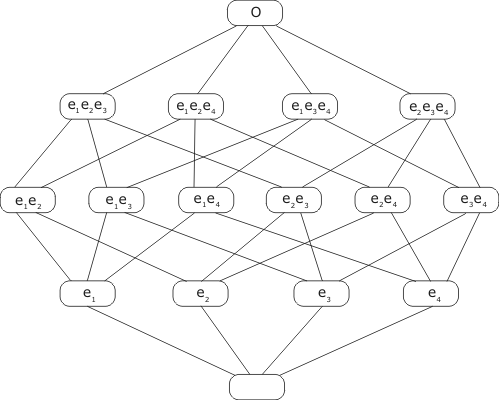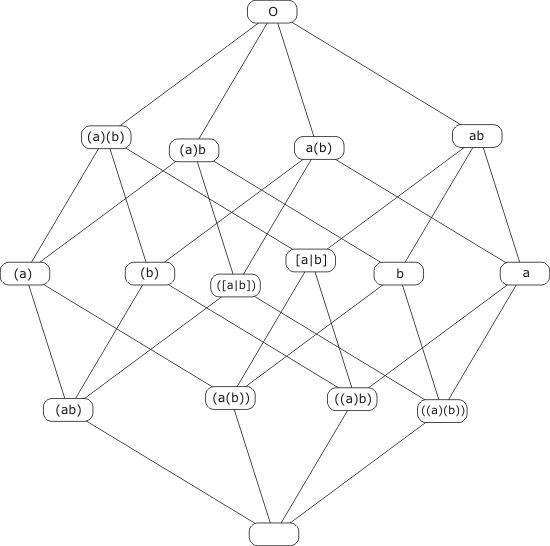### The number of elements in a Finite Boolean Algebra

If a is dominated by b (that is, ab=b), but a is not equal to b, we say that a follows b and that a is a follower of b. The 'following' of an element x is the number of followers of x.

In this page we use the notation a≤b to signify that a is dominated by b, and a<b to signify that a is dominated by b, but is not equal to b.

We note that _ has no followers; O has the whole of the Boolean Algebra (let's call it B), except itself, following it; and all other elements (if there are any) are followed by _ , at least.

An element that has only one follower (namely _ ) we call an atom.

A first criterion of atomicity
An element e is an atom iff for any x in B, if x is dominated by e (i.e. if ex=e) then x=_ or x=e.

For xe=e says the same as x≤e, that is, x is either a follower of e, or equal to e. But if e is atomic, the only follower of e is _; therefore, x is either e or _ . Conversely, the criterion says that e has only one follower, implying that e is an atom.

A second criterion of atomicity
An element e is an atom iff for all x in B, (e)x = (e) or O.

Proof: We prove that the first criterion implies the second, and vice-versa. Now, in any case, ((e)x) is dominated by e, for

e((e)x) = e(( )x) = e(O) = e.

Therefore, if e satisfies the first criterion, ((e)x) must be either _ or e ; and so

(e)x = O or (e) .

Therefore, the second criterion is satisfied. Conversely, in any case

x = ( (ex) ((e)x) )

by each way; therefore, if e satisfies the second criterion,

x = ((ex)) or x = ((ex) e).

This is true for all x; now suppose x is dominated by e (so ex=e). Then

x = ((e)) = e or x = ((e)e) = _ , Q.E.D

Lemma If a follows b and b follows c then a follows c.

Proof Certainly a≤c; it remains to show that a≠c. For if a was equal to c, then a≥c; but as b≥a as well, we would have b≥c, contrary to the premise that b follows c.

Lemma Suppose that b has a finite following. Then if a<b, a has fewer followers than b.

Proof Every follower of a is also a follower of b; but in addition a itself is a follower of b (but not of itself).

From now on let us assume that B itself is finite.

Lemma Suppose that x, an element of B, has more than one follower; then among the followers of x is an atom.

Proof Pick a non-void follower x1 of x. If x1 has a following of one, then x1 is an atom and we are done. Otherwise, pick a non-void follower x2 of x1. Again, if x2 has a following of one, then x2 is an atom and we are done. Otherwise, pick a non-void follower x3 of x2; and so on... But the succesive xi have fewer and fewer followers. Since x itself has a finite number of followers, we must eventually arrive at an xi which is an atom.

Suppose we have found a few atoms e1, e2, e3 up to en.

Lemma If P, the combination of e1, e2, e3, ... en is not equal to O, then there exists another atom f not in the set e1...en.

Proof The premise implies that (P) is non-void, so it has at least one follower. If only one, then (P) is an atom; otherwise, (P) has a follower f which is an atom. In either case we have an atom f which is ≤(P). But if f were one of the set e1...en, we would also have f≤P. But f≤(P) and f≤P imply (f)(P)=O and (f)P=O, respectively; so by each way

f = ((f)P) ((f)(P)) = _ ,

a contradiction. Therefore f is not one of the set e1...en .

Corollary If e1...en is a complete set of atoms, containing all the atoms in B, then P=e1e2..en=O.

Converse If e1...en is a set of atoms, whose product P = e1e2..en is equal to O, then there are no other atoms in B.

Proof Suppose P equalled O, and that f was another atom, not equal to any of the ei. Then, for all i,

(f)ei = (f) or O

(by the second criterion of atomicity). But the second possibility would imply that f was a follower of ei, which is impossible. So in every case (f)ei = (f). Then

$\dpi{130} (f) = \prod_{i=1 \dots n} (f)e_i = (f) \prod_{i=1 \dots n}e_i = (f)O = O ,$

implying f = _ , which is a contradiction.

Theorem If A = {e1, e2, ..en} is a complete set of atoms, than any x in B can be expressed as a combination of some of the atoms in A. Thus

$\dpi{130} x = \prod_{i\in X}e_i$

where X is a subset of N={1,2,..n}.

Proof By the corollary to the lemma above, and letting P stand for the product of all the atoms, and using distribute, we have

x = ((x)) = ((x)(P)) = ( (x)( ((e1)) ((e2)) ... ((en)) ) ) = ((e1)(x)) ((e2)(x)) ... ((en)(x));

but each (ei)(x) is either (ei) or O, by the second criterion of atomicity ; so x is a product of factors, each one of which is ei or _ . If we define X as the set of integers i in N such that (ei)(x)=(ei) then

$\dpi{130} x = \prod_{i\in X}e_i .$

Thus any x in B can be expressed as a product of atoms; conversely, any product of atoms is a member of B. Moreover, it can never be the case that two different products of atoms give rise to the same element of B. Thus

$\dpi{130} \prod_{i\in X}e_i = \prod_{i\in Y}e_i \; \text{iff} \; X=Y.$

Proof As a preliminary, we note that for all i and j in N,

(ej)ei = (ej) or O,

by the atomicity of ej. If the latter, ej is a follower of ei, which implies that i=j. Therefore the first equality will hold iff i≠j, the second iff i=j.

Now, suppose j is in the subset X but not in Y. Then

$\dpi{130} (e_j) \prod_{i\in Y}e_i = \prod_{i\in Y}(e_j)e_i = (e_j) .$

On the other hand,

$\dpi{130} (e_j) \prod_{i\in X}e_i = O ;$

therefore the two products cannot be equal. A similar contradiction would arise if j were in Y but not in X. Therefore, if the atomic products are equal, X and Y must be the same subset.

If there are n atoms, the number of subsets of N is 2n, and this is the number of distinct atomic products. But these correspond to the elements of B in a one-to-one fashion, therefore B has 2n elements.

Example Suppose B is the set of equivalence classes of CL-expressions involving the letters a and b. Then the following expressions are all atoms:

(ab), (a(b)), ((a)b), ((a)(b)) .

This can be proved using the second criterion of atomicity. To start with, let E = (ab); then, for all expressions F (not involving any letters other than a and b)

(E)F = abF = ab Fab = ab_ or abO = ab or O = (E) or O.

(Here Fab means the expression that results when _ is substituted in F for both a and b: so Fab is an arithmetic expression which may be reduced to _ or O. The reasoning here is similar to that used in the proof of the Case Analysis Lemma.) Thus E is indeed an atom. Very similar proofs apply to the other three expressions. The product of the four atomic expressions is

(ab) (a(b)) ((a)b) ((a)(b)) = (a)a = O,

by each way. They are therefore all the atoms, and the number of elements in B must be 24 = 16. (Of course we knew all this already, but it is just as well to see it from another angle.)

In any Boolean algebra with 16 elements, the scheme of dominance is as follows:In the example under consideration, this translates into:We have used the notation [a|b] for the equivalence expression of a and b

(a(b))((a)b) .

This figure has suggested to some people that the 16 elements could be envisaged as the 16 vertices of a 4-dimensional cube or 'hypercube'.